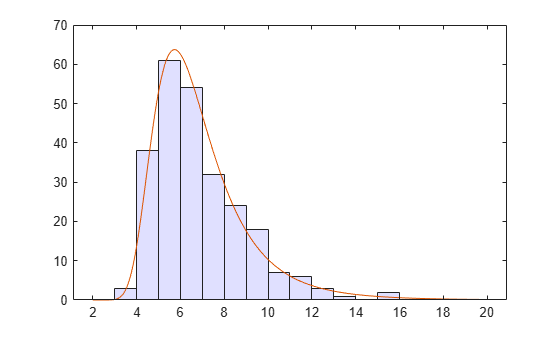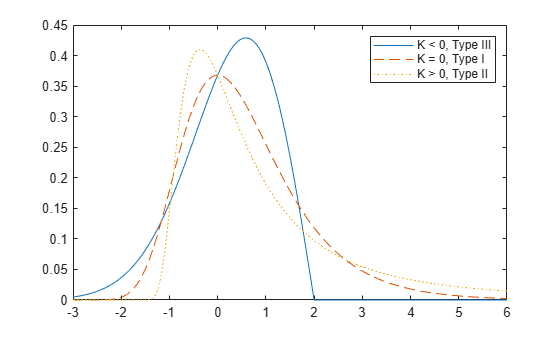## Generalized Extreme Value Distribution

### Definition

The probability density function for the generalized extreme value distribution with location parameter µ, scale parameter σ, and shape parameter `k``0` is

`$y\text{​}\text{​}\text{\hspace{0.17em}}\text{\hspace{0.17em}}=\text{​}\text{\hspace{0.17em}}f\left(x|\text{k},\mu ,\sigma \right)=\text{​}\text{​}\text{​}\text{​}\text{​}\text{​}\text{\hspace{0.17em}}\left(\frac{1}{\sigma }\right)\mathrm{exp}\left(-{\left(1+\text{k}\frac{\left(x-\mu \right)}{\sigma }\right)}^{-\frac{1}{\text{k}}}\right){\left(1+\text{k}\frac{\left(x-\mu \right)}{\sigma }\right)}^{-1-\frac{1}{\text{k}}}$`

for

`k > 0` corresponds to the Type II case, while ```k < 0``` corresponds to the Type III case. For `k = 0`, corresponding to the Type I case, the density is

`$y\text{​}\text{​}\text{\hspace{0.17em}}\text{\hspace{0.17em}}=\text{​}\text{\hspace{0.17em}}f\left(x|0,\mu ,\sigma \right)=\text{​}\text{​}\text{​}\text{​}\text{​}\text{​}\text{\hspace{0.17em}}\left(\frac{1}{\sigma }\right)\mathrm{exp}\left(-\mathrm{exp}\left(-\frac{\left(x-\mu \right)}{\sigma }\right)-\frac{\left(x-\mu \right)}{\sigma }\right)$`

### Background

Like the extreme value distribution, the generalized extreme value distribution is often used to model the smallest or largest value among a large set of independent, identically distributed random values representing measurements or observations. For example, you might have batches of 1000 washers from a manufacturing process. If you record the size of the largest washer in each batch, the data are known as block maxima (or minima if you record the smallest). You can use the generalized extreme value distribution as a model for those block maxima.

The generalized extreme value combines three simpler distributions into a single form, allowing a continuous range of possible shapes that includes all three of the simpler distributions. You can use any one of those distributions to model a particular dataset of block maxima. The generalized extreme value distribution allows you to “let the data decide” which distribution is appropriate.

The three cases covered by the generalized extreme value distribution are often referred to as the Types I, II, and III. Each type corresponds to the limiting distribution of block maxima from a different class of underlying distributions. Distributions whose tails decrease exponentially, such as the normal, lead to the Type I. Distributions whose tails decrease as a polynomial, such as Student's t, lead to the Type II. Distributions whose tails are finite, such as the beta, lead to the Type III.

Types I, II, and III are sometimes also referred to as the Gumbel, Frechet, and Weibull types, though this terminology can be slightly confusing. The Type I (Gumbel) and Type III (Weibull) cases actually correspond to the mirror images of the usual Gumbel and Weibull distributions, for example, as computed by the functions `evcdf` and `evfit` , or `wblcdf` and `wblfit`, respectively. Finally, the Type II (Frechet) case is equivalent to taking the reciprocal of values from a standard Weibull distribution.

### Parameters

If you generate 250 blocks of 1000 random values drawn from Student's t distribution with 5 degrees of freedom, and take their maxima, you can fit a generalized extreme value distribution to those maxima.

```blocksize = 1000; nblocks = 250; rng default % For reproducibility t = trnd(5,blocksize,nblocks); x = max(t); % 250 column maxima paramEsts = gevfit(x)```
```paramEsts = 1×3 0.1185 1.4530 5.8929 ```

Notice that the shape parameter estimate (the first element) is positive, which is what you would expect based on block maxima from a Student's t distribution.

```histogram(x,2:20,'FaceColor',[.8 .8 1]); xgrid = linspace(2,20,1000); line(xgrid,nblocks*... gevpdf(xgrid,paramEsts(1),paramEsts(2),paramEsts(3)));```### Examples

#### Compute the Generalized Extreme Value Distribution pdf

Generate examples of probability density functions for the three basic forms of the generalized extreme value distribution.

```x = linspace(-3,6,1000); y1 = gevpdf(x,-.5,1,0); y2 = gevpdf(x,0,1,0); y3 = gevpdf(x,.5,1,0); plot(x,y1,'-', x,y2,'--', x,y3,':') legend({'K < 0, Type III' 'K = 0, Type I' 'K > 0, Type II'})```Notice that for `k > 0`, the distribution has zero probability density for `x` such that $x<-\sigma /k+\mu$.

For `k < 0`, the distribution has zero probability density for $x>-\sigma /k+\mu$.

For `k = 0`, there is no upper or lower bound.